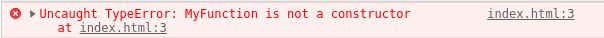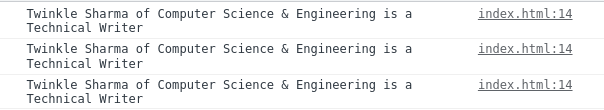GeeksforGeeks App
Open AppBrowser
Continue

# What is the arrow function, and how to create it ?

Function in any programming language is the basic building block to create and combine the related bits of code. Every programming language provides certain kinds of practices to write any function. The arrow function syntax is one of the most used and efficient ones to create a function in JavaScript.

How to create an arrow function: To write the arrow function, simply create any variable it can be const, let, or var but always do prefer const to avoid unnecessary problems. And then do assign the function code to the variable that’s it. So from now, you can call that function by writing the parenthesis in front of that variable. With arrow function syntax, we consider a function as an object and assign the definition to some variable. Following is the syntax of the arrow function:

```const myFunction = (param1, param2,
.... paramN) => { // function code }
const myFunction = (param) => { // function code }
or
const myFunction = param => { // function code }
const myFunction = param => { return param*param }
or
const myFunction = param => param*param```

We can omit the {} parenthesis when there is only one statement and the javascript considers that statement as a return value, also there is no need to write parenthesis () when there is only one parameter. The arrow function can’t contain the line break between the (params) and the arrow =>, Also there should not be any space between the = and > characters.

Example 1: In this example, we will create a program to add two numbers first using the normal function and then using the arrow function.

Using normal function:

## Javascript

 `function` `myFunction() {``    ``let a = 5, b = 4;``    ``return` `a + b;``}``console.log(myFunction());`

Using arrow function:

## Javascript

 `const myFunction = () => {``    ``let a = 5, b = 4;``    ``return` `a + b;``}``console.log(myFunction());`

Output: In the arrow function, we don’t write the function keyword, so it is necessary to assign it to some kind of variable like here we have assigned to a constant variable named myFunction.

`9`

Example 2: In this example, we will create a function of single expressions using both normal and arrow functions one by one.

Using normal function:

## Javascript

 `function` `myFunction(num) {``    ``return` `num * num;``}``console.log(myFunction(4));`

Using arrow function:

## Javascript

 `const myFunction = num => num * num;``// Equivalent to const myFunction = (num) =>{ return num*num; }` `console.log(myFunction(4));`

Output: When we have only one return expression in the function, arrow syntax provides an easier way to write. We can drop the parenthesis of the parameter and also the return statement along with code blocks braces.

`16`

Limitations of Arrow Functions: Earlier we have seen how easily we can create a function with the arrow syntax now it’s time to look at some limitations also because they will not work similarly to normal functions in several situations.

No binding of this keyword: It cannot be used as a method because of not having a binding of this keyword. The arrow function contains the lexical this instead of their own. The value of this will be based upon the scope in which they are defined. So the arrow function is defined in the window scope hence this will refer to the window object instead of the object in which the function is been written. There doesn’t exist any property or method with ‘num’ hence undefined will be printed.

## Javascript

 `var` `obj = {``    ``num: 10,``    ``myFunc: () => { console.log(``this``.num) }``}` `obj.myFunc();`

Output:

`undefined`

Explanation: The normal function contains this which refers to the object to which it belongs, and hence the function belongs to the obj object and property num exists inside it, so will be printed successfully.

Arrow functions can’t be used as constructors.

Example: This example shows that an arrow function can’t be used as a constructor.

## Javascript

 `var` `MyFunction = () => { };``var` `instan = ``new` `MyFunction();`

Output:The call, apply, bind methods don’t work with arrow functions: Below is the general recall for concepts of these methods with the example of the traditional function, and later we will see that it won’t work well with arrow functions.

• call() Method: This method is used to call any function for an object, and inside the function, this will refer to the object being passed.
• apply() Method: It also works similarly to the call method. Instead of passing arguments separately here, we pass an array of arguments.
• bind() Method: This method is also used to call any function for an object but it creates another copy of that function by binding the object which can be used later.

Example: This example shows the use of the call(), apply(), and bind method in Javascript.

## Javascript

 `let studentObj1 = {``    ``studentName: ``"Twinkle Sharma"``,``    ``branch: ``"Computer Science & Engineering"``}` `function` `showDetails(position) {``    ``console.log(`\${``this``.studentName} of \${``this``.branch} is a \${position}`)``}` `showDetails.call(studentObj1, ``"Technical Writer"``);` `showDetails.apply(studentObj1, [``"Technical Writer"``]);` `const myFunction = showDetails.bind(studentObj1);` `myFunction(``"Technical Writer"``);`

Output: Because this refers to the scope in which the arrow function is defined means the window This is the reason why the call, apply, and bind methods don’t work with the arrow function. Hence we can only access the window with the help of the arrow function. And as the properties student name and branch don’t exist in the window object undefined will be printed.My Personal Notes arrow_drop_up
Related Tutorials